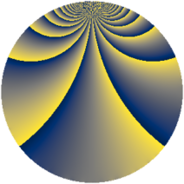# Properties

 Label 637.2.wLevel $637$ Weight $2$ Character orbit 637.w Rep. character $\chi_{637}(92,\cdot)$ Character field $\Q(\zeta_{7})$ Dimension $336$ Newform subspaces $2$ Sturm bound $130$ Trace bound $1$

# Related objects

## Defining parameters

 Level: $$N$$ $$=$$ $$637 = 7^{2} \cdot 13$$ Weight: $$k$$ $$=$$ $$2$$ Character orbit: $$[\chi]$$ $$=$$ 637.w (of order $$7$$ and degree $$6$$) Character conductor: $$\operatorname{cond}(\chi)$$ $$=$$ $$49$$ Character field: $$\Q(\zeta_{7})$$ Newform subspaces: $$2$$ Sturm bound: $$130$$ Trace bound: $$1$$ Distinguishing $$T_p$$: $$2$$

## Dimensions

The following table gives the dimensions of various subspaces of $$M_{2}(637, [\chi])$$.

Total New Old
Modular forms 396 336 60
Cusp forms 372 336 36
Eisenstein series 24 0 24

## Trace form

 $$336 q - 56 q^{4} - 8 q^{5} + 16 q^{6} - 6 q^{7} - 12 q^{8} - 36 q^{9} + O(q^{10})$$ $$336 q - 56 q^{4} - 8 q^{5} + 16 q^{6} - 6 q^{7} - 12 q^{8} - 36 q^{9} - 20 q^{10} + 10 q^{11} + 50 q^{12} - 2 q^{13} + 48 q^{14} + 8 q^{15} - 56 q^{16} - 6 q^{17} - 20 q^{18} + 48 q^{19} + 16 q^{20} - 28 q^{21} + 16 q^{22} + 14 q^{23} - 56 q^{24} - 84 q^{25} - 6 q^{26} + 6 q^{27} - 42 q^{28} + 48 q^{29} - 52 q^{30} + 56 q^{31} + 40 q^{32} - 44 q^{33} + 56 q^{34} - 46 q^{35} - 16 q^{36} - 16 q^{37} - 38 q^{38} + 116 q^{40} - 16 q^{41} + 92 q^{42} - 36 q^{43} - 60 q^{44} + 82 q^{45} + 60 q^{46} - 28 q^{47} - 136 q^{48} - 28 q^{49} - 60 q^{50} - 44 q^{51} - 6 q^{52} + 8 q^{53} - 84 q^{54} + 98 q^{55} - 32 q^{56} - 22 q^{57} + 60 q^{58} - 48 q^{59} + 152 q^{60} - 48 q^{61} - 74 q^{62} - 86 q^{63} - 116 q^{64} + 110 q^{66} - 32 q^{67} - 168 q^{68} - 76 q^{69} - 104 q^{70} + 22 q^{71} + 66 q^{72} - 52 q^{73} - 116 q^{74} + 134 q^{75} + 54 q^{76} - 88 q^{77} + 50 q^{78} - 52 q^{79} + 132 q^{80} + 44 q^{81} - 140 q^{82} - 10 q^{83} + 110 q^{84} - 68 q^{85} + 48 q^{86} - 76 q^{87} + 54 q^{88} - 18 q^{89} - 142 q^{90} - 4 q^{91} - 52 q^{92} + 108 q^{93} + 44 q^{94} - 92 q^{95} - 10 q^{96} - 36 q^{97} - 104 q^{98} + 104 q^{99} + O(q^{100})$$

## Decomposition of $$S_{2}^{\mathrm{new}}(637, [\chi])$$ into newform subspaces

Label Dim $A$ Field CM Traces $q$-expansion
$a_{2}$ $a_{3}$ $a_{5}$ $a_{7}$
637.2.w.a $162$ $5.086$ None $$3$$ $$0$$ $$-4$$ $$-15$$
637.2.w.b $174$ $5.086$ None $$-3$$ $$0$$ $$-4$$ $$9$$

## Decomposition of $$S_{2}^{\mathrm{old}}(637, [\chi])$$ into lower level spaces

$$S_{2}^{\mathrm{old}}(637, [\chi]) \cong$$ $$S_{2}^{\mathrm{new}}(49, [\chi])$$$$^{\oplus 2}$$